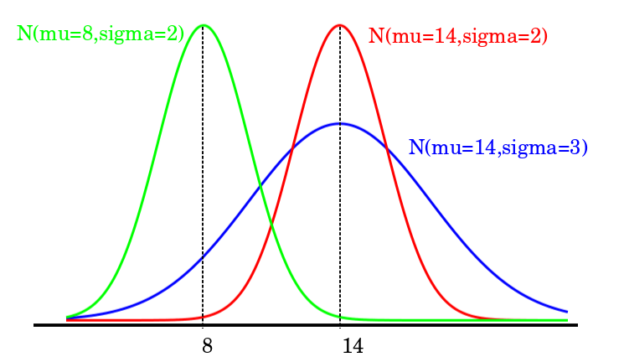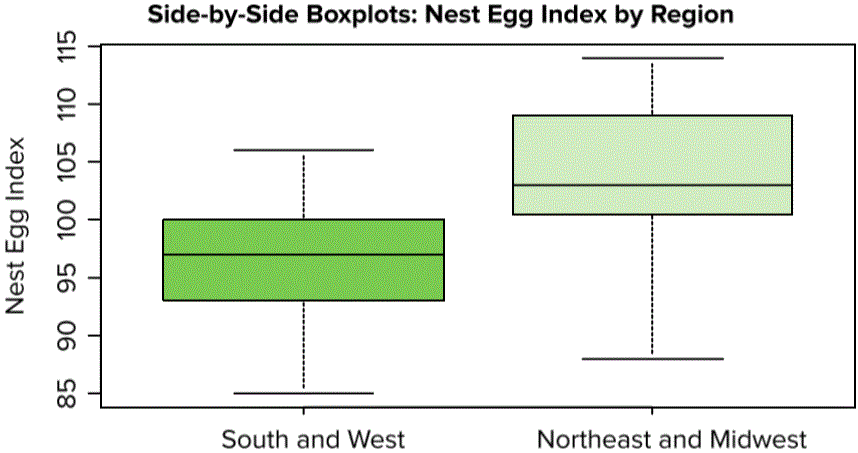Are you more of a visual learner? Check out our online video lectures and start your statistics course now for free!Image: “Comparing normal distribution.” by Joxemai – Own work. License: CC BY-SA 3.0

## Big Picture

The big picture of distributions comprises:

• Graphical display, which helps in getting an idea of the shape of the graph
• Summary statistics, which aids in making an idea about the center and spread of the data sets.

## Comparing Groups

### Histogram

A histogram is a graphical method for displaying the shape of a distribution. It is particularly useful when there are many observations.  The distribution of multiple groups can be compared by analyzing the histogram of two data. The histogram analysis of multiple groups can be compared and analyzed by observing the following features of histograms.

• Shape: whether the shapes of distribution are alike or different from each other.
• Spread distribution: whether one histogram shows more spread out than the other one.
• Center of distribution: is the center of distributions has shown at different places on both histograms.
• Unusual feature: unusual features refer to gaps (areas of the distribution where there are no observations) and outliers.

Example: The datasets can be compared by using histograms so suppose the three top-selling smartphones popular on the market are LG G5, Samsung S7, and iPhone 7 plus. The specifications of the three phones are as follows:

 Samsung S7 iPhone 7 plus LG G5 Size (length×width×depth) 142 mm × 73 mm × 8.1 mm 124 mm × 59 mm × 7.6 mm 131 mm × 79 mm × 8.1 mm Weight 145 g 112 g 143 g Material Plastic Aluminum Plastic Screen Resolution 432 ppi 326 ppi 423 ppi Camera 16 MP 8 MP 13 MP Fingerprint Sensor Yes Yes No Water Resistant Yes No No Price (without the contract) 650 \$ 650 \$ 450 \$

Table: Specifications of Samsung S7, iPhone 7 plus, and LG G5

In order to find out which smartphone is the best and has the most unique features, a study has been conducted. A survey of 80 people was conducted including both males and females including questions of how much hours per day they spend on social networking through their wanted smartphones. The response of both males and females include:

Females

 3.5 4 3.7 3.3 3.8 3.1 4.1 3.3 3.1 4.1 3.4 4.1 3.8 3.4 3.7 3.8 3.3 4.1 3.7 3.9 3.8 3.3 4.5 3.9 3.4 3.8 3 3.9 3.7 4.1 4 4.2 3 3.5 4.2 4.1 3.9 3.4 4.3 3

Males

 2.7 2.4 1.5 2.7 3 3.3 3.1 2.4 2 2.3 2.3 3 2.5 1.9 2.8 3 2 2.6 2.9 3.2 2.8 2 2.7 2.7 2.7 3.1 3.2 2.6 3.1 2.6 2.6 2.7 2.9 2.3 3.2 2.4 2.5 1.6 2.8 2.8

Table: Response from Males and Females

The data taken from two sets can be compared by using a histogram.

The comparison of two datasets has shown that, on average, females spend 3.6 to 3.9 hours per day on social media networking. On the other hand, males spend 2.7 hours per day for interacting with their social networks using their smartphones.

### Box plots

In order to compare two groups using box plots, a side by side comparison is required. Box plots give a sense that how the shapes of box plots differ in terms of skewness and symmetry. One of the troubles in using box plots for a comparison of different groups is that it hides modes.

Box plots provide basic information about a distribution. For example, a distribution with a positive skew would have a longer whisker in the positive direction than in the negative direction. A larger mean than median would also indicate a positive skew.

It is helpful for comparison of spreads of distribution of two or more groups. Box plots should be placed side by side in the same plot for comparison of different groups. In order to understand the comparison of different groups by use of box plot, an example is given as follows:

With parallel box plots, data from two groups are displayed on the same chart, using the same measurement scale. Let’s compare a medical study where a treatment group was supplied medicine for a cure for cold symptoms. The box plots will show the number of days each medical group has to report symptoms to seniors.

The box plots have shown unusual features, such as outliers or gaps. Both box plots have skewed towards the right side, whereas the skewness in the box plot of the treatment group is prominent and sharp.

The skewness in the treatment group indicates that a slight less response of patients has been noticed in this group, as compared to the control group. As the data shown in box plots, in the treatment group the cold symptoms lasted till 2 weeks or 14 days, giving it a range = 13.

In the control group, the same lasted 3 to 17 days, giving it a range = 14. The median recovery time of the treatment group has shown 5 days, whereas 9 days for the control group indicating a positive effect on patient recovery.”

Box plot comparison is helpful in the determination of spread and center. In terms of shape, it is not reliable.

## Comparing Distributions

Distributions can be compared by using box plots through side by side comparison.

### Side by side comparison

Box plots can be used to compare the distribution of two groups. Box plots help in making an analysis of how the shapes of two box plates differ from each other in terms of symmetry and skewness. Box plots are not good at the identification of modes, but it is helpful in the identification and comparison of the spread between two distributions.

Box plots are good at portraying extreme values and are especially good at showing differences between distributions. However, many of the details of a distribution are not revealed in a box plot, and to examine these details one should create a histogram and/or a stem and leaf display.“Side-by-Side Boxplots: Nest Egg Index by Region” Image created by Lecturio

## Outliers

An outlier is an abnormal or distant observation in a population. An outlier may occur due to a variance in the measurement of data or experimental errors. Normally, experimental data error is excluded from data set so we are left with outlier caused by variance in measurement. If a population has a heavy-tailed distribution, it may also cause outliers in data.

Outlier can be classified into three main types:

Point outlier: If an individual data point can be considered anomalous with respect to the rest of the data, then the datum is termed as a point outlier. This is the simplest type of outlier, and it is the focus of the majority of research on outlier detection.

Contextual outlier: If an individual data instance is anomalous in a specific context (but not otherwise), then it is termed as a contextual (conditional) outlier. The notion of a context is induced by the structure of the data set, and has to be specified as a part of the problem formulation.

Collective outlier: If a collection of data points is anomalous with respect to the entire data set, it is termed as a collective outlier. The individual data points inside the collective outlier may not be outliers by themselves alone, but their occurrence together as a collection is anomalous. Collective outliers can occur only in data sets in which data points are somehow related.

### Impact of outlier on data description

An outlier ranges far from mid of the data point or mean position. It is normally located on extreme positions, i.e. higher or much lower point. Outliers located at a much higher position than mid-point of the data cause inflation of mean and reduction in mean when located at a much lower position to the mean point. Outlier included in a calculation based on the reasonable expectation of finding outlier in a population.

### Caution

Outliers are required to be investigated considerately in order to get accurate results from a data set. Outliers are valuable information during the process of the data gathering and reading process in a biological research process. Outliers are bad points which can contaminate a data set; they should be eliminated from the data.

## Data Transformation

Data transformation is the process of making distributions more systematic and putting on a different scale in the form of a different unit as compared to the previous one. If data is skewed, it may be difficult for the researcher to summarize distributions through the use of center and spread. It can also create problems for finding out whether the outliers are the extreme values identified in the chart or graph, or if it is just a part of a stretched tail.

Log transformation

It aims at making the distribution of a variable more symmetric and more linear. It helps in achieving normality of a data set.

The log transformation can be used to make highly skewed distributions less skewed. This can be valuable both for making patterns in the data more interpretable and for helping to meet the assumptions of inferential statistics.

The comparison of the means of log-transformed data is actually a comparison of geometric means. This occurs because, as shown below, the anti-log of the arithmetic mean of log-transformed values is the geometric mean.

Table 1 shows the logs (base 10) of the numbers 1, 10, and 100. The arithmetic mean of the three logs is

(0 + 1 + 2)/3 = 1.

The anti-log of this arithmetic mean of 1 is

101 = 10

Which is the geometric mean:

(1 x 10 x 100).3333 = 10.

Logarithms.

 X Log10(X) 1 10 100 0 1 2

Therefore, if the arithmetic means of two sets of log-transformed data are equal, then the geometric means are equal.

A histogram can be used to assess the linearity of data. It aims at creating uniformity in the spread of several groups, despite the difference between their centers.

Side by side box plots can be used for this assessment. Data transformation aims at making the form of scatterplot more linear and to avoid thickening around the line in a plot by spreading the scatterplot evenly.

### Log transformation

In some cases, the transformation of data through the ladder of powers does not aid properly in fixing the curvature of a scatterplot. Logarithmic transformations are helping to sort such issues.

• X-axis: x y-axis: In(y) – exponential transformation is suitable for data values which tend to increase by percentage.
• X-axis: In (x), y-axis: y-Logarithmic model is helpful when scatter plot decline both at left and right side of the plot.
• X-axis: y-axis: In (y) – power transformation – when above-mentioned types of logarithmic transformations are not helpful, this transformation aids.

Equalizing the spread between two groups

The comparison of the two groups is normally based on an assumption that the variance of one group is equal to the variance of the other group. Logarithmic transformation is helpful in equalizing the spread between two groups of data sets.

## Issues with Comparison of Distribution

Some of the major issues associated with distribution comparisons include:

1. Use of inconsistent scales

Transformation of independent or dependent both variables should be avoided. It can cause inconsistency of scales. Two variables should always be compared in the same units for the correct result.

2. Labeling plots

The plot should be labeled clearly and accurately. If labeling is not done properly, it may create ambiguity.

3. Beware of outliers

Outliers should be identified and removed timely in order to avoid any kind of variance in data. If outliers are not removed, summarize the data twice with and without outliers.

Learn. Apply. Retain.
Your path to achieve medical excellence.
Study for medical school and boards with Lecturio.